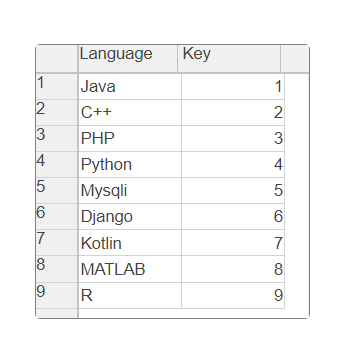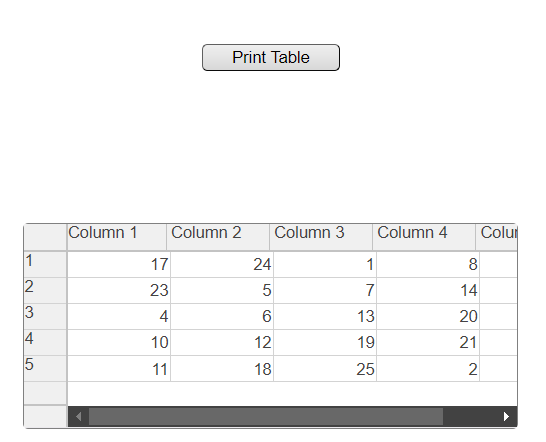GFG App
Open AppBrowser
Continue

GUI tables in MATLAB are graphical user interface that allow users to display and manipulate tabular data.They are used to create interactive applications that require data to be displayed in a table format. GUI tables in MATLAB typically consist of columns and rows , with each column representing a variable or field and each row representing a record or instance of the data. The user can interact with the table by selecting cells, sorting and filtering data, editing cell values, and performing other operations. Graphical User Interface in MATLAB can be customized to suit specific application requirements , including changing column widths, hiding or showing columns, changing cell color and fonts and setting callbacks for user events , they are commonly used in scientific and engineering applications, financialanalysis, and data visualization.

In this article, we will create  GUI based Tables in MATLAB. GUI means is graphical user interface which means interface which display on screen and user can see in this article we will create a GUI tables by using MATLAB.

### Using the ‘uitable’ Function

This is the very easiest way to create GUI-based table,  first we created a figure using fig and than we defined column names and also data like languages and key and then we filled data by define new data and then we created  uitable by using uitableand then we define the table width, height, position by using table.position method.

Example 1:

## Matlab

 `% Code` `% Code` `% Create a figure window` `fig = figure();`   `% Define column names and data` `colNames = {``'Language'``, ``'Key'``};` `data = {``'Java'``, 1; ` `    ``'C++'``, 2;``'PHP'``,3; ``'Python'``, 4;``'Mysqli'``,5;` `    ``'Django'``, 6;``'Kotlin'``,7;``'MATLAB'``, 8;``'R'``,9};`   `% Create a uitable in the figure window` `table = uitable(fig, ``'Data'``, data, ``'ColumnName'``, colNames);`   `% Set table properties` `table.Position = [80 80 200 200];`

Output :### Scrolling Table

In this method we created a table which we can scroll upto left to right for see data for this we are using uitable function in which we declare data magic(5) and we first declare column name like column1, column2 and so on and then we created an push btn and then we print our table by using another function printtable.this way we can create scrolling table and also we can change the table width height by changing the position value in out code.

Example 2:

## Matlab

 `% Code` `function` `guiTable`   `    ``% Create the figure and table` `    ``fig = figure(``'Position'``,[100 100 400 300]);` `    ``t = uitable(fig,``'Data'``,magic(5),``'ColumnName'``,` `    ``{``'Column 1'``,``'Column 2'``,``'Column 3'``,` `    ``'Column 4'``,``'Column 5'``},` `    ``'Position'``,[20 20 360 150]);`   `    ``% Create the push button` `    ``btn = uicontrol(``'Style'``,``'pushbutton'``,``'String'``,` `    ``'Print Table'``,``'Position'``,[150 280 100 20],` `    ``'Callback'``,@printTable);`   `    ``% Define the push button callback function` `    ``function` `printTable(~,~)` `        ``disp(get(t,``'Data'``))` `    ``end`   `end`

Output:### Application of GUI Based Table in MATLAB

Here are five different applications of GUI-based tables in MATLAB:

1. Data Analysis
2. Experiment Control.
3. Financial Applications
4. Education
5. Quality Control

My Personal Notes arrow_drop_up